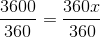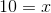# High School Math : How to find the percentage of a sector from an angle

## Example Questions

### Example Question #1 : Sectors

Two pizzas are made to the same dimensions. The only difference is that Pizza 1 is cut into pieces at 30° angles and Pizza 2 is cut at 45° angles. They are sold by the piece, the first for $1.95 per slice and the second for$2.25 per slice. What is the difference in total revenue between Pizza 2 and Pizza 1?

$2.70$5.40

–$2.70$0

–$5.40 Correct answer: –$5.40

Explanation:

First, let's calculate how many slices there are per pizza. This is done by dividing 360° by the respective slice degrees:

Pizza 1: 360/30 = 12 slices

Pizza 2: 360/45 = 8 slices

Now, the total amount made per pizza is calculated by multiplying the number of slices by the respective cost per slice:

Pizza 1: 12 * 1.95 = $23.40 Pizza 2: 8 * 2.25 =$18.00

The difference between Pizza 2 and Pizza 1 is thus represented by: 18 – 23.40 = –\$5.40

### Example Question #1 : Circles

What percentage of a full circle is the following sector? (Round to the nearest tenth of a percent.)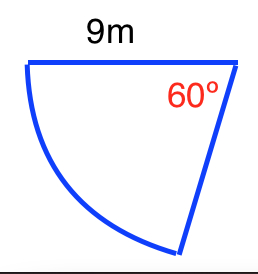Note: The figure is not drawn to scale.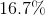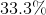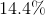Explanation:

In order to find the percentage of a sector from an angle, you need to know that a full circle is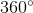.

Therefore, we can find the percentage by dividing the angle of the sector byand then multiplying by 100: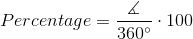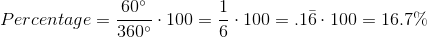### Example Question #2 : Circles

A sector of a circle contains a center angle that is 36 degrees. What percentage of the circle is the sector?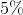Explanation:

Proportions can be used to determine the percentage. Letequal the percentage comprised of the sector.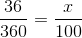Cross multiply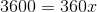Solve for x.